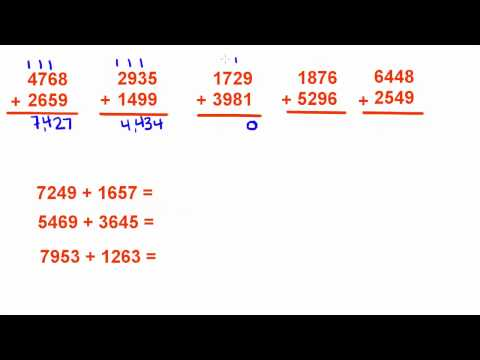# thenewboston► Play all

# Basic Math Tutorials Playlist

• thenewboston
• 10 videos
• 54,493 views
• Last updated on Jun 14, 2014
thenewboston Official Basic Math Tutorials Playlist!

Loading...

•  Basic Math Tutorial - 1 - Addition of Whole Numbers 3:59 Basic Math Tutorial - 2 - Addition with Decimals 5:06 Basic Math Tutorial - 3 - Addition with Perimeters 2:47 Basic Math Tutorial - 4 - Addition of Money 5:22 Basic Math Tutorial - 6 - Subtracting with Decimals 6:43 Basic Math Tutorial - 7 - Fill in the Missing Measurement 3:14 Basic Math Tutorial - 8 - Subtracting Money 6:28 Basic Math Tutorial - 9 - Write the Equivalent for Each Measurement 3:33 Basic Math Tutorial - 10 - Find the Missing Side 2:01 Basic Math Tutorial - 11 - Word Problems with Time 6:33
to add this to Watch Later

### Add to

Loading playlists...